## General Chemistry

In all the chapters we covered so far, we dealt with chemical reactions where the changes occur as a result of electron redistributions. For example, the reaction between sodium metal and chlorine gas is an example of a redox reaction where the chlorine being more electronegative takes the electron from the metal thus forming a cation and anion which are then boned by an ionic bond: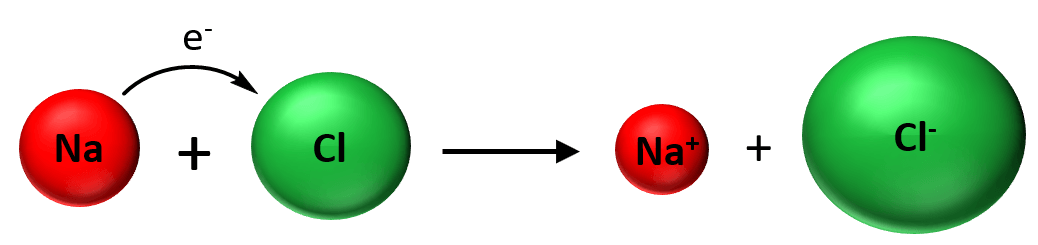In the context of today’s topic, the key here and in any other chemical reaction is that the elements do not convert into other elements. This can be seen in acid-base, precipitation, redox, and in any other chemical reaction:

2HNO3 + Ba(OH)2 → Ba(NO3)2 + 2H2O(l)

3C2O22- + Cr2O72- + 14H+(aq) → 6CO+ 2Cr3+ + 7H2O(l)

2AgNO3(aq) + CaCl2(aq) → 2AgCl(s) + Ca(NO3)2(aq)

The pairing, and the bonding change but there are no new elements appearing in the products.

# Nuclear Reactions

Contrary to chemical reactions, nuclear reactions are associated with changes in the atomic nuclei. This process is also known as transmutation. Now, when one element changes into another, very often tremendous amounts of energy is released. In the basis of nuclear reactions is the instability of large atomic nuclei. All the elements with Z > 83 (Z is the atomic number) exhibit some type of nuclear activity such as spontaneously emitting particles and/or electromagnetic radiation. This is called radioactivity/nuclear decay/radioactive disintegration/nuclear disintegration and such atoms are said to be radioactive.

Before we get into some details of nuclear reactions, recall the following definitions from the atomic structure.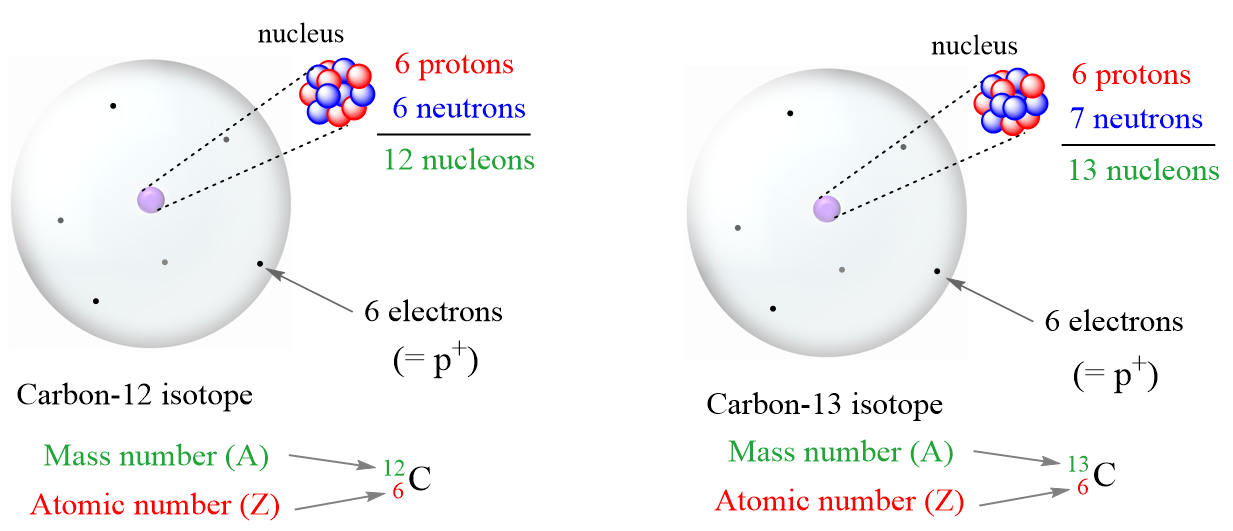• Proton (p or p+) – a subatomic particle with a positive electric charge of 1+.
• Neutron (n or no) – a neutral subatomic particle slightly heavier than proton.
• Electron (e) – a subatomic particle with a negative electric charge of 1-.
• Atomic number (Z) the number of protons in an atom. Always the same for the given element
• Mass number (A) – the sum of the number of neutrons and protons
• Every atom has an equal number of electrons and protons, so atoms have no net
• electrical charge.
• Isotopes – atoms with the same number of protons (atomic number) but different numbers of
• Because of a different number of neutrons, isotopes have different mass numbers.
• Nucleons – a general term for both protons (p) and neutrons (n)
• Nuclide – the nucleus of a given isotope

The main types of radioactivity are alpha (α) decay, beta (β) decay, gamma (γ) radiation, positron emission, and electron capture: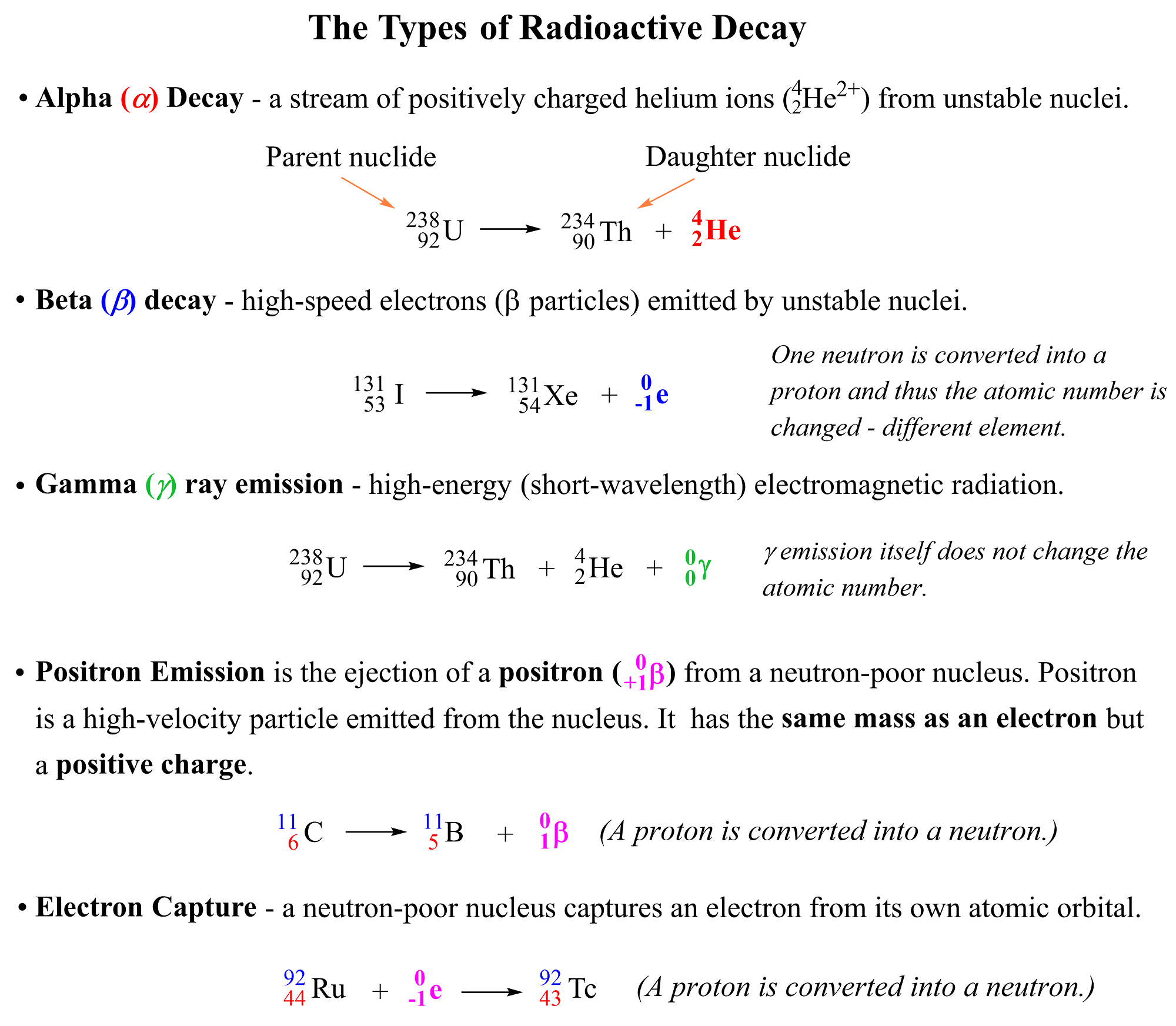Notice again that in all reactions above, the starting element is converted into another one by releasing certain particles or radiation. So, let’s discuss each of these radioactive processes one by one.

# Alpha (α) Decay

Alpha (α) decay occurs when an unstable nucleus loses a particle composed of two protons and two neutrons. These are called alpha particles symbolized as α, 24α, or 24He2+ which are identical to the helium-4 nuclei.

For example, 23892U atoms decompose to 23490Th atoms emitting alpha particles: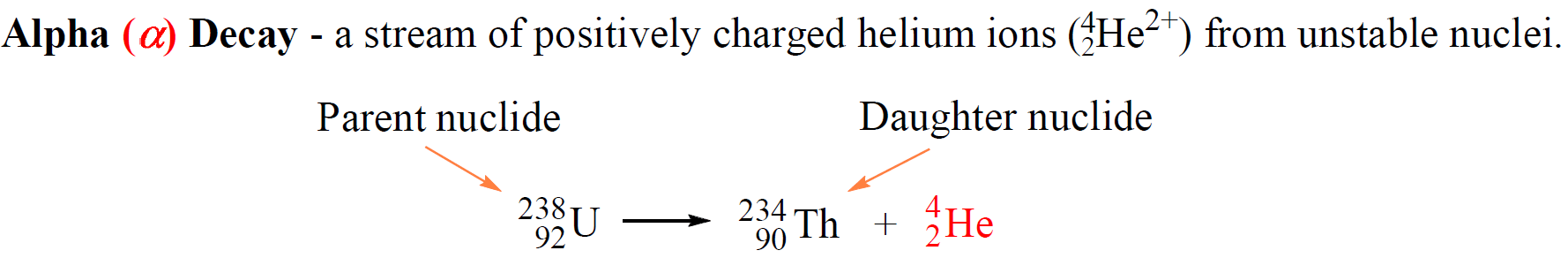The original atom, shown on the left side of the equation is the parent nuclide, and the product of the decay is the daughter nuclide. In this case, uranium-238 is the parent nuclide and it converts into the daughter nuclide, thorium-234.

So, what is the reason that uranium transforms thorium? Remember, the identity of the element is given based on its number of protons. Now, alpha particles consist of two protons and two neutrons and emitting an alpha particle changes the number of protons in in the nucleus transforming the element into a different element.

Nuclear reactions are represented by nuclear equations which are always balanced. The sum of the mass numbers and the sum of the atomic numbers on both sides are equal. For the decomposition of uranium, the sum of the protons on the right side (90 + 2) is equal to the atomic number of uranium, and the sum of the mass numbers of Th and α (234 + 4) is equal to the atomic mass of the 23892U isotope.# Predicting the Product of Alpha Decay

Because we know that in alpha decay, the mass number of the parent nuclide must decrease by four, and the atomic number decreases by 2 units, we can determine the identity of the daughter nuclide. For example, identify the daughter nuclide for the alpha decay of 224Ra.

Solution: We can start by writing the symbol of 224Ra on the left side of the nuclear equation and adding an alpha particle on the right side of the equation. Meanwhile, look up the atomic number of Ra in the periodic table, and write it as well since it will help identify the daughter nuclide:

22488Ra → X + 42He

To determine the number of protons of the daughter nuclide, we subtract 2 (the two protons of the alpha particle) from 88 (the atomic number of Ra):

X (atomic number) = 88 – 2 = 86

Therefore, the atomic number of the daughter nuclide is 86, and using the periodic table, we determine that it is radon (Rn):

22488Ra → 86Rn + 42He

At this point, we only need to determine the mass number of the specific Rn isotope that is formed in this reaction.

22488Ra → ?86Rn + 42He

This, again, is determined based on the fact that the sum of the mass numbers must be balanced on both sides of the nuclear equation. Therefore, the mass number of Rn is the difference between those of 22488Ra and 42He:

M (86Rn) = M (22488Ra) – M (42He)

M (86Rn) = 224 – 4 = 220

Therefore, the balanced equation for the alpha decay of 22488Ra can be written as:

22488Ra → 22086Rn + 42He

# Beta (β) Decay

Beta (B) decay is the emission of an electron from an unstable nucleus. For example, the conversion of the 13153I isotope to the 13154Xe isotope is a beta decay: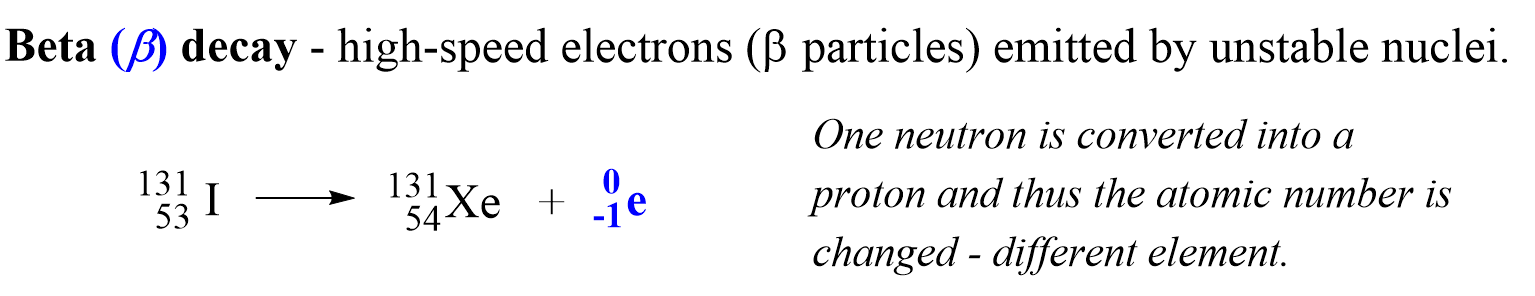Before addressing the important features of the beta decay, there is one question you might be wondering about: How is the electron coming from the nucleus if the latter contains only protons and neutrons?

And the answer is, yes, there is no free electron in the nucleus, and it is rather generated when a neutron, in an unstable nucleus, changes into a proton:

10n →11p + 0-1e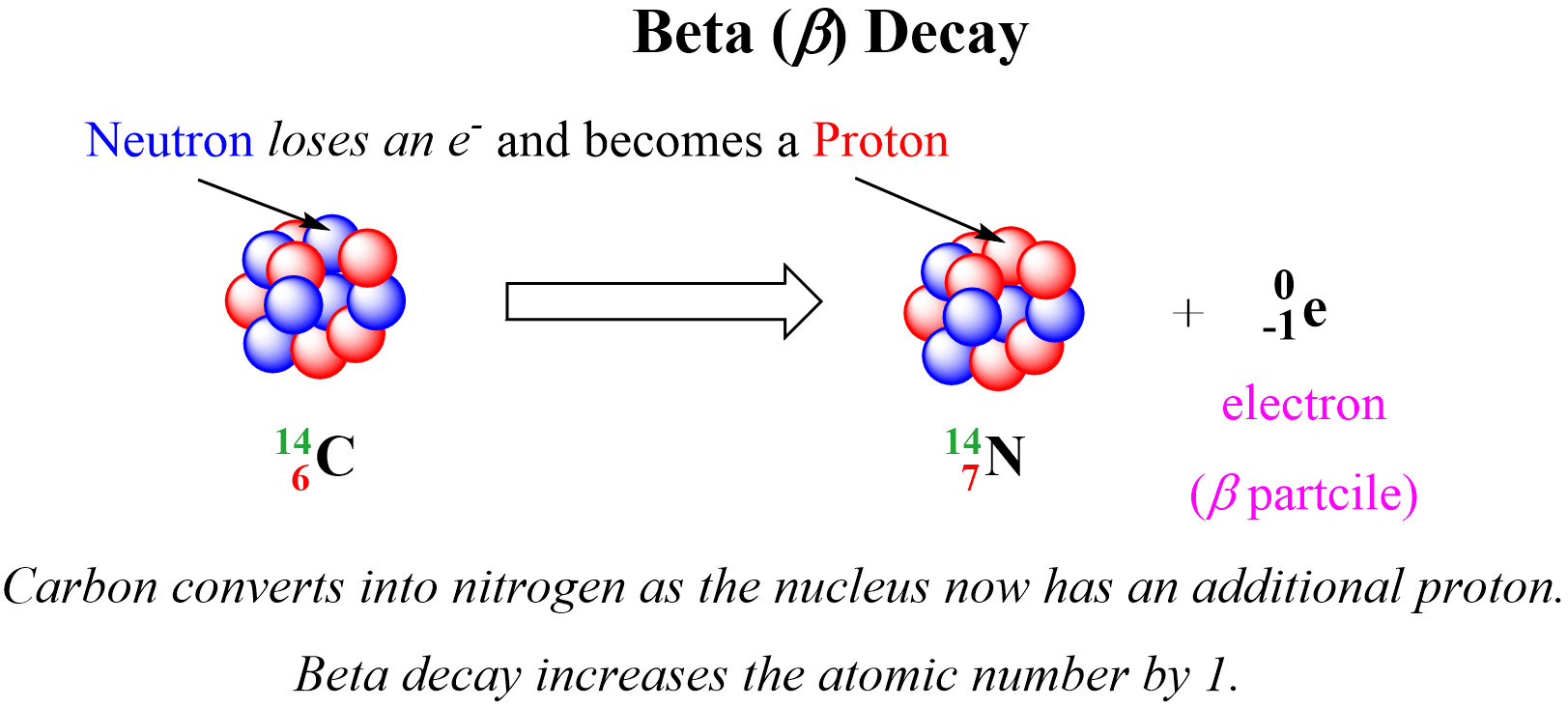In the example above, one of the neutrons in the 14C nucleus is converted into a proton because losing a negatively charged particle with negligible charge converts a neutral particle into a positively charged particle. Therefore, there is a new atom formed with 7 protons which corresponds to the 14N isotope. So, for a general pattern, we can say that beta radiation increases the atomic number by 1:Notice again that the mass number does not change during a beta decay since the mass of the electron is negligible and does not contribute to the atomic mass. Just like in alpha decay, the nuclear equation of beta decay is balanced as the sum of the mass numbers and the atomic numbers are equal on both sides of the equation.

Gamma radiation is different from the alpha and beta decays because it is an emission of high-energy γ photons also called γ rays. So, gamma radiation is an emission of light with very short wavelength and high frequency. Remember, we saw in earlier chapters that light/photons are emitted from atoms in an excited state where electron(s) fall from higher energy states to the ground or a lower energy state: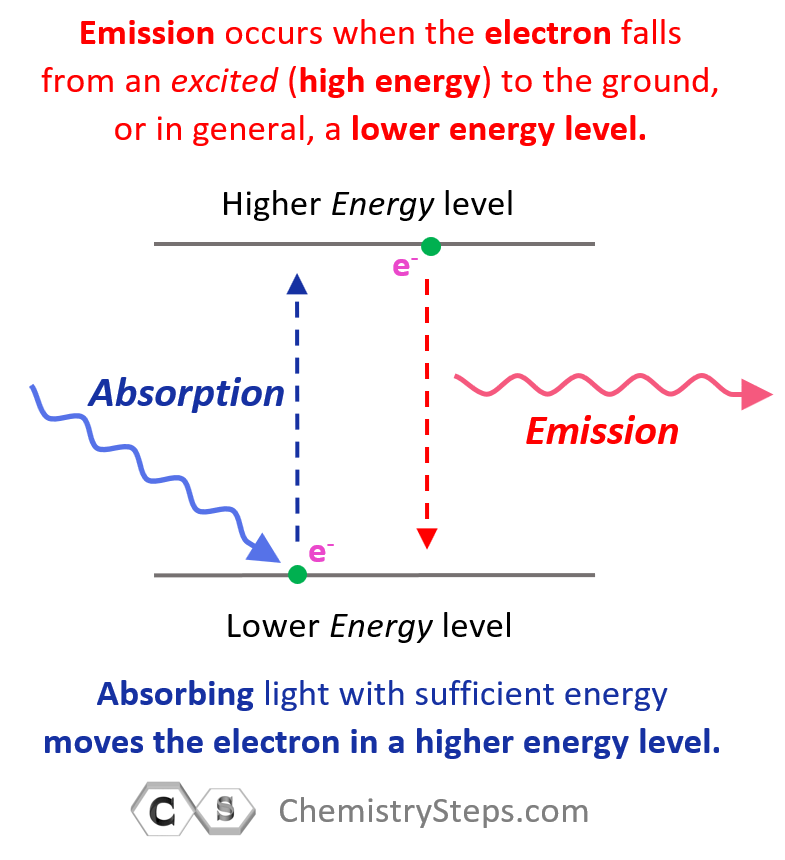Now, gamma rays are emitted from nuclei in excited states the description of which is beyond the scope of our discussion. If the gamma radiation is not accompanied by other types of emission that we discussed above, then the atomic number and mass number are not changed.

However, if the gamma radiation occurs in conjunction with other types of radiation, then the conversion of the parent nucleus is defined based on the type of radiation. For example, the alpha decay of 238U is also accompanied by gamma radiation.

23892U → 23490Th + 42He + 00γ

Depending on the nucleus, several γ photons of different energies can be emitted from an excited nucleus as it returns to the ground state. For example, Marie Curie discovered that the Polonium-215 nuclide converts into Pb-211 isotope by emitting gamma arrays and alpha particles:

21584Po → 21182Pb + 42He + several 00γ

Alpha and beta decays are so often associated with gamma radiation that it is often left out of nuclear equations. It is simply presumed that chemists know that there are gamma rays associated with the given radiation.

In some cases, however, the parent nuclide converts through gamma radiation into an intermediate unstable nuclide which subsequently emits a particle.

9943Tc → 99m43Tc → 9944Ru + 0-1β + several 00γ

The mTc notation indicates a metastable state of the technetium nuclide which emits gamma photons. This is often emitted from the equation and the net transformation is shown as:

9943Tc → 9944Ru + 0-1β + several 00γ

# The Power of Different Radiations

Now that we talked about three common radioactive decays, let’s also mention their power and penetrating ability.

The reference point for the power of radiation is its ionizing power which is the to ionize other molecules and atoms.

Among all types of radioactivity, the highest ionizing power is characteristic of alpha radiation. However, because of their larger size, alpha particles have the lowest penetrating power. They can be shielded by a sheet of paper while the gamma radiation can only be blocked by a thick layer of lead.Beta radiation is intermediate between the alpha particles and gamma photons. They are much smaller than alpha particles and therefore, have lower ionizing power. The smaller size on the other hand gives them a higher penetrating power.

The common analogy for comparing the ionizing and penetration power of radioactivity is the comparison of a bus (alpha particle), a sedan (beta particle), and a motorcycle (gamma rays). The bus will have the greatest power; however, it can be stopped by many obstacles making the road narrower. Gamma rays are motorcycles; they have the lowest ionizing power but the greatest penetration power. All types of radioactivity are dangerous to living organisms when penetrating a cell. Alpha particles, therefore, may not impose danger if outside of the body since they can be stopped even by the molecules in the air. Ingesting radioactive nuclide emitting alpha particles can certainly cause a lot of danger to the body. The hazard of radioactivity can be extremely severe, and if the subject interests you, you can search for the consequence of radioactivity in nuclear bombs, failure of nuclear reactors, and even poisoning by radioactive elements.

# Positron Emission

Positron Emission is the ejection of a positron ( 0+1β) from a neutron-poor nucleus. Positron is a high-velocity particle emitted from the nucleus and it has the same mass as an electron, but the opposite charge, it is the antiparticle of the electron.

We can visualize positron emission as shown below: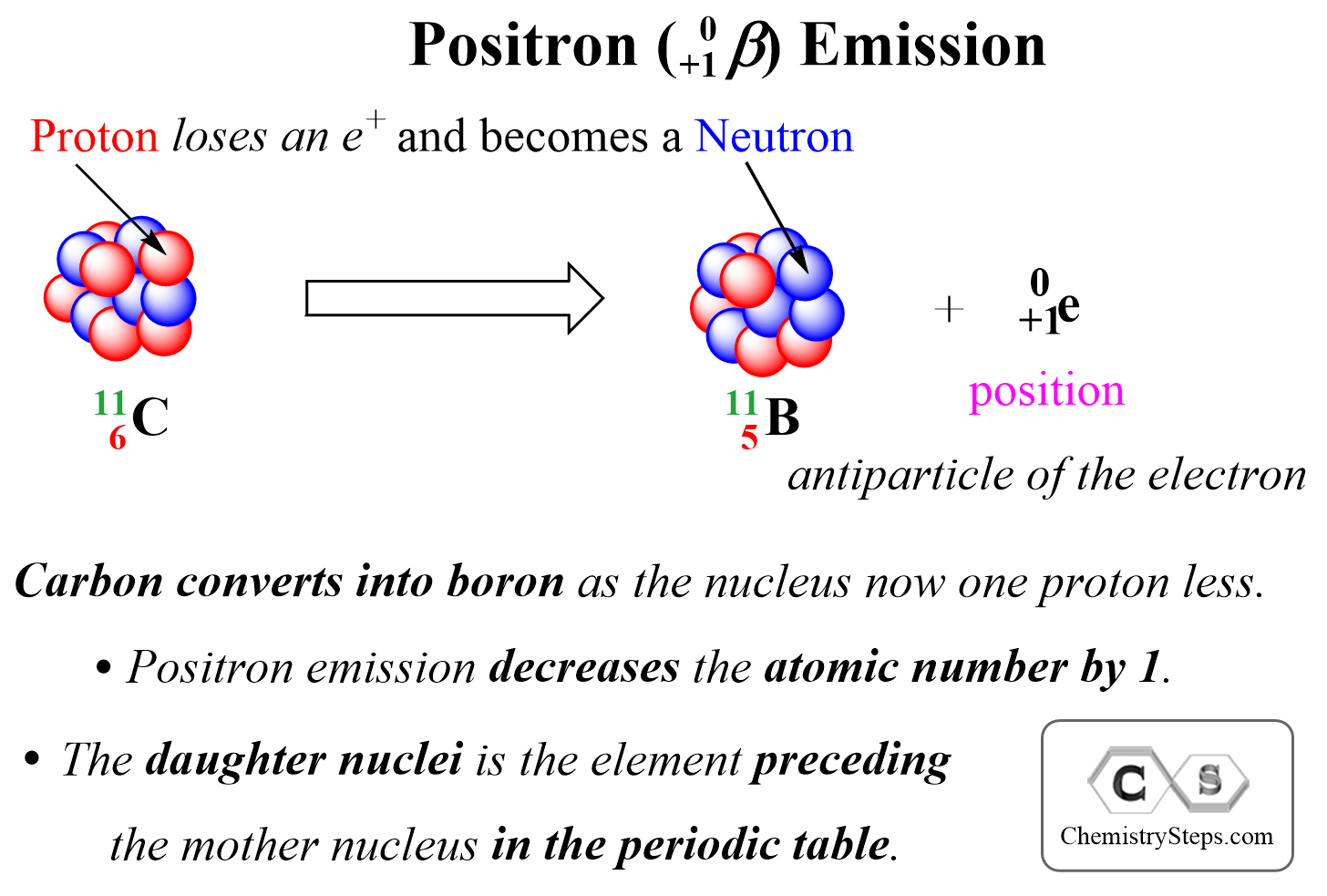Positron emission decreases the atomic number by 1, and therefore, the daughter nucleus is the element preceding the mother nucleus in the periodic table.

For example, if we are asked to write the nuclear equation for the position emission of the 3015P isotope, we can right away figure out that the daughter element is going to be Si as it comes before P in the periodic table:

3015P → 3014Si + 0+1β (or 0+1e)

# Electron Capture

Electron capture occurs when a neutron-poor nucleus absorbs an own electron from an inner shell atomic orbital. Like positron emission, the electron capture decreases the atomic number by 1 as one proton is converted into a neutron.

For example, Ru-92 undergoes electron capture, converting into Tc-92:

9244Ru  + 0-1e →  9243Tc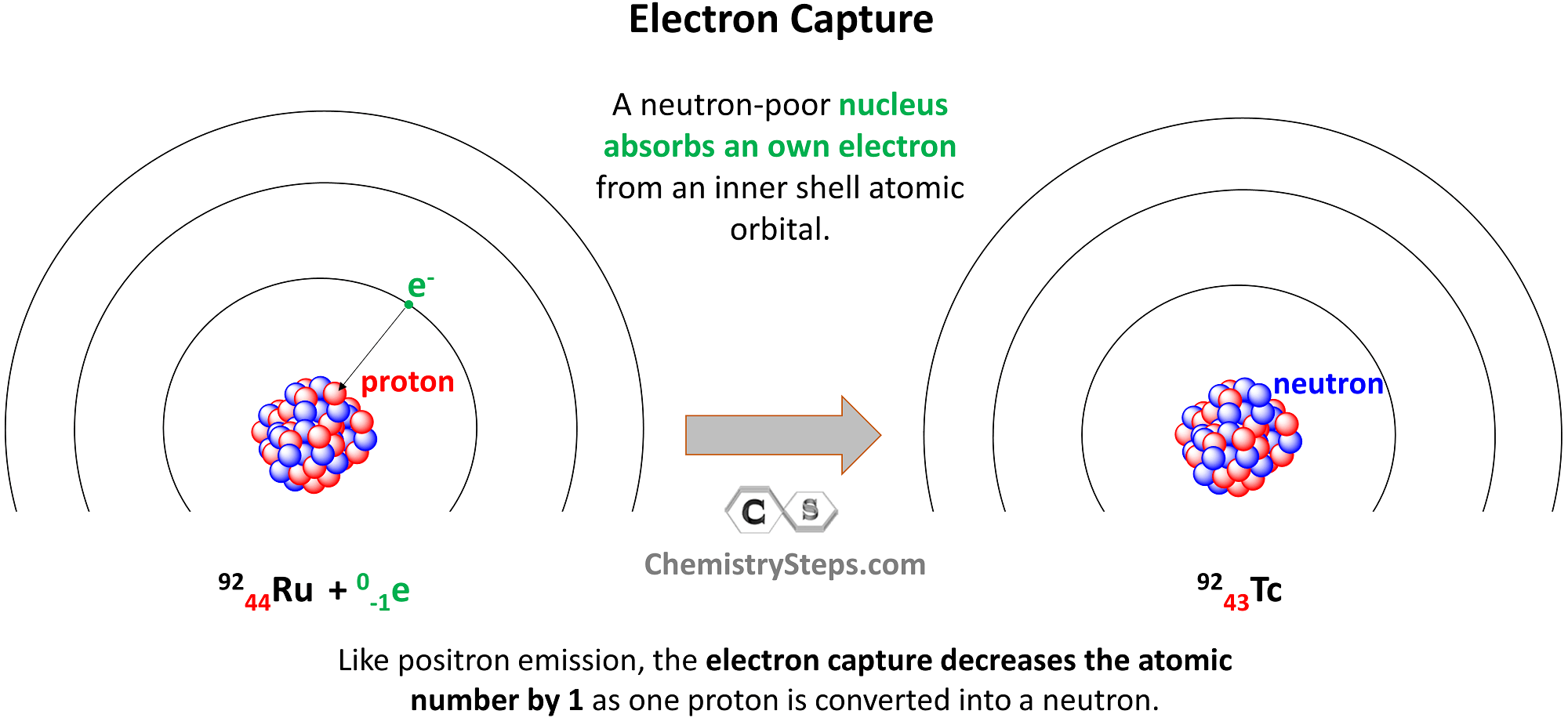Let’s put together a summary of radioactive processes and how they affect the number of protons and neutrons in the nucleus:Out of all the radioactive processes discussed today, it is only the alpha decay that decreases the number of protons and neutrons and, as a result, the atomic mass of the daughter nuclide also decreases

In position emission and electron capture, the number of protons decreases by one and that of neutrons increases by one because one proton is converted into a neutron. Therefore, an isotope of the element preceding the mother nuclide in the periodic table is formed which has the same atomic mass.

In beta decay, a negatively charged particle (electron) is emitted from a neutron which converts into a proton, and therefore, the daughter nuclide is an isotope of the element that follows the mother nuclide in the periodic table.

Gamma radiation by itself does not change the number of protons or neutrons unless accompanied by another type of radioactive decay. So, unless there is another type of radioactive decay, gamma radiation does not alter the nuclide, and therefore, the mass number stays the same as well.

1686023026